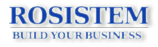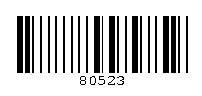First barcoding website from Romania!
 Home | About Us | Solutions | Tutorials | Barcode Education | Biometric Education | Contact Us
Barcode Education » MSI Code
 IntroductionMSI Data Corporation introduced MSI from the original Plessey Code. Therefore, MSI is also known as Modified Plessey. It is mainly used in retail business. MSI/Plessey is a fixed-length, non-self-checking symbology. Most applications still support MSI only for backward compatibility, because MSI/Plessey is based on Pulse-Width Modulation technology. MSI requires start/stop characters. MSI is used to encode numeric data and check digit is required to add at the end of the data. Variants MSI is sometimes called Plessey Code or MSI/Plessey. Structure of a Code 11 barcode A typical MSI/Plessey barcode has the following structure: 1. A start character 2. Message encoded 3. Check Digit 4. A stop character Check Digit Calculation MSI uses one or two check digits, which may be calculated in a number of different ways. As such, it is really up to the software application to implement and check the check digit(s). The most common methods of calculating MSI check digits are: Modulo 10 To calculate the modulo 10 checksum digit, use the following steps: 1. Create a new number using every other digit from the original code such that the right-most digit of the new number is the right-most digit of the old number. For example, in the barcode above the data we encoded was "8052". In this case, the "new number" is 02. 2. Take the new number calculated in step 1 and multiply it by 2. In this case, 02 * 2 is 4. 3. Add the digits of the value calculated in the previous step (4), and add it to the digits that were not used in step 1 to form the new number. In our example, this would be 4 + 8 + 5 = 17. The "4" comes from step 2, the 8 and 5 come from the "8052" and are the digits that weren't used to form the new number in step 1. If the result from step 2 were, for example, 123, then we'd add 1 + 2 + 3 = 6 (plus the digits that weren't used from step 1). 4. Do a modulo 10 calculation on the result of step 3. In this case, 17 modulo 10 = 7. 5. The check digit is the value which, added to the result in step 4, equals 10. In this case, we must add 3 to 7 to get 10-so the check digit is 3.